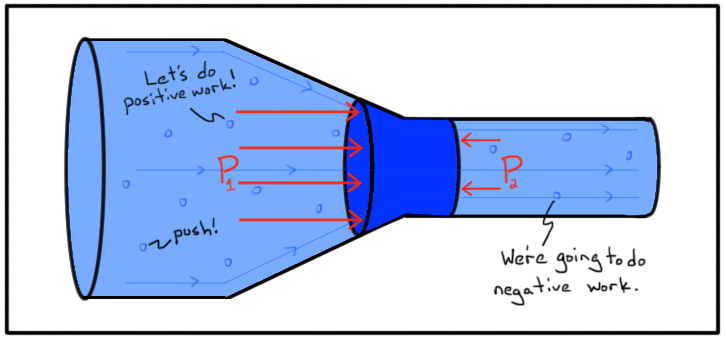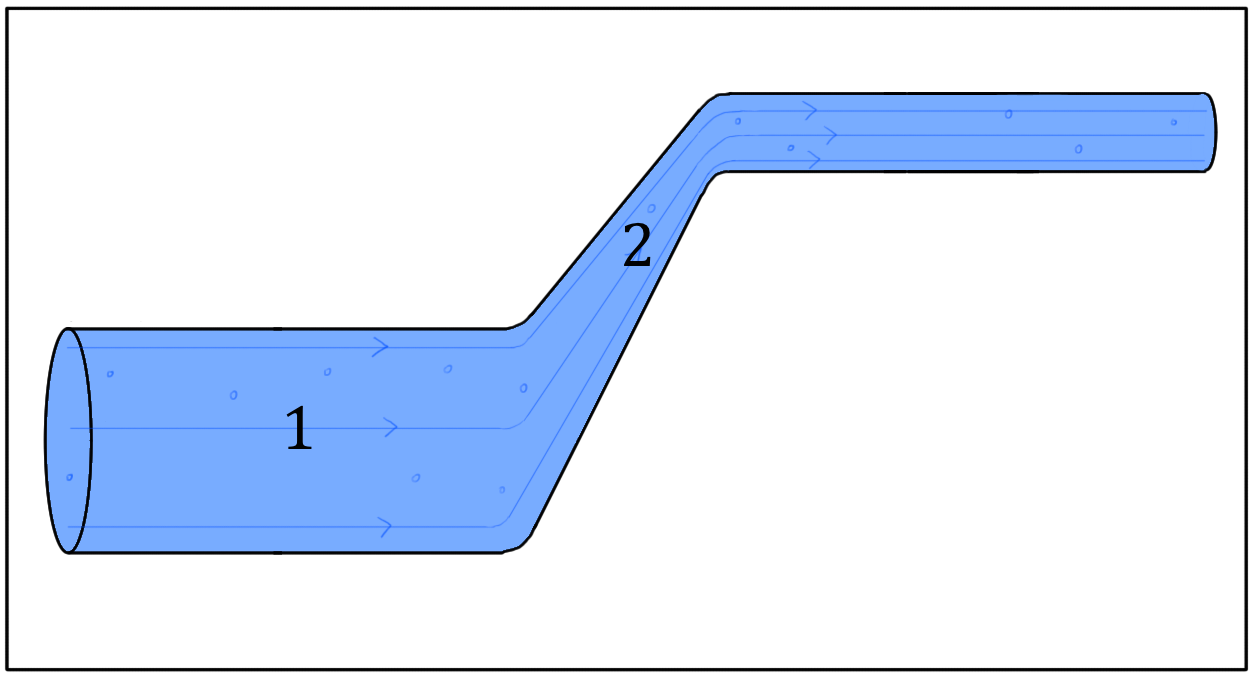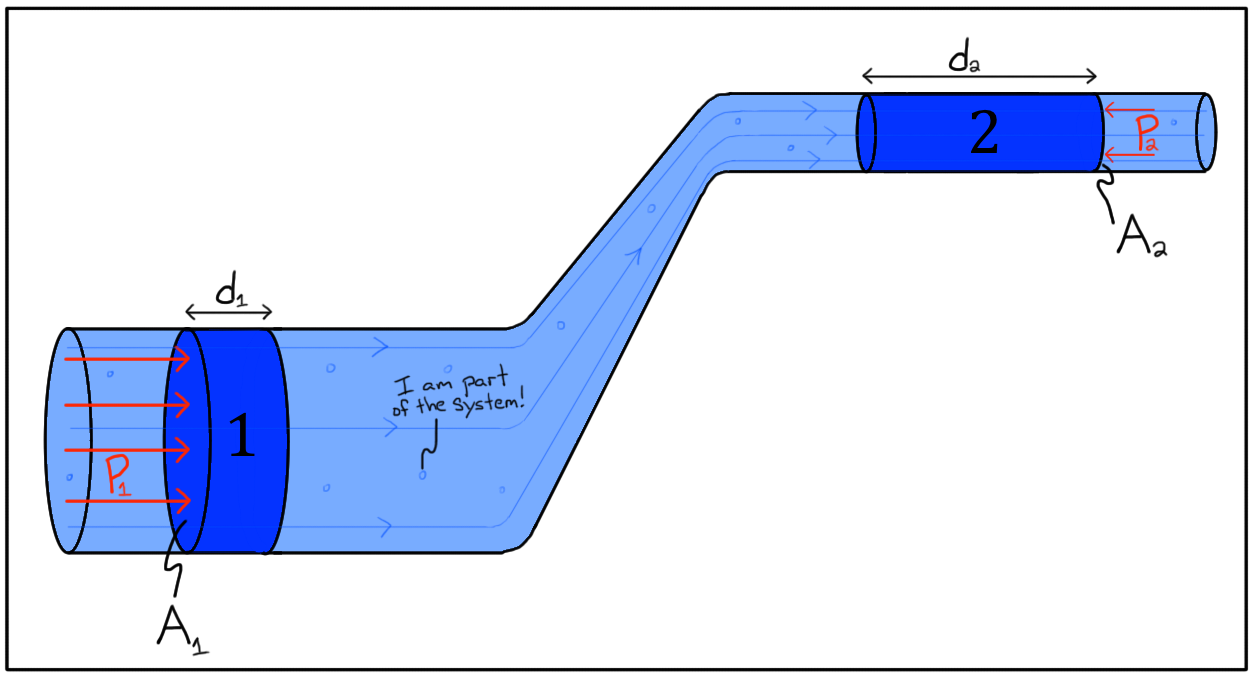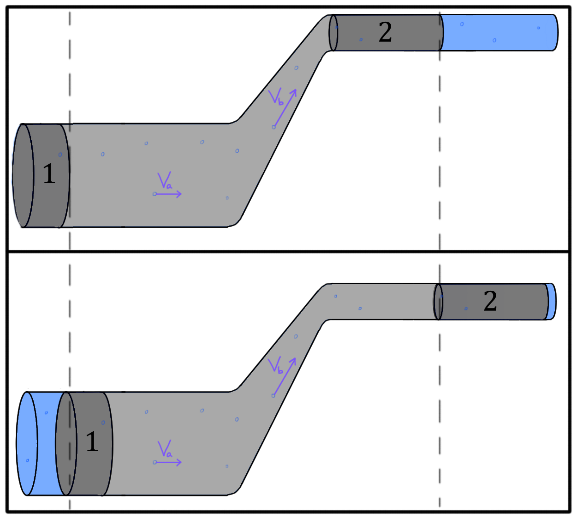## What is Bernoulli's equation?

This equation will give you the powers to analyze a fluid flowing up and down through all kinds of different tubes.

## What is Bernoulli's principle?

Bernoulli's principle is a seemingly counterintuitive statement about how the speed of a fluid relates to the pressure of the fluid. Many people feel like Bernoulli's principle shouldn't be correct, but this might be due to a misunderstanding about what Bernoulli's principle actually says. Bernoulli's principle states the following,
Bernoulli's principle: Within a horizontal flow of fluid, points of higher fluid speed will have less pressure than points of slower fluid speed.
So within a horizontal water pipe that changes diameter, regions where the water is moving fast will be under less pressure than regions where the water is moving slow. This sounds counterintuitive to many people since people associate high speeds with high pressures. But, we'll show in the next section that this is really just another way of saying that water will speed up if there's more pressure behind it than in front of it. In the section below we'll derive Bernoulli's principle, show more precisely what it says, and hopefully make it seem a little less mysterious.

## How can you derive Bernoulli's principle?

Incompressible fluids have to speed up when they reach a narrow constricted section in order to maintain a constant volume flow rate. This is why a narrow nozzle on a hose causes water to speed up. But something might be bothering you about this phenomenon. If the water is speeding up at a constriction, it's also gaining kinetic energy. Where is this extra kinetic energy coming from? The nozzle? The pipe?
The only way to give something kinetic energy is to do work on it. This is expressed by the work energy principle.
W, start subscript, e, x, t, e, r, n, a, l, end subscript, equals, delta, K, equals, start fraction, 1, divided by, 2, end fraction, m, v, start subscript, f, end subscript, start superscript, 2, end superscript, minus, start fraction, 1, divided by, 2, end fraction, m, v, start subscript, i, end subscript, start superscript, 2, end superscript
So if a portion of fluid is speeding up, something external to that portion of fluid must be doing work it. What force is causing work to be done on the fluid? Well, in most real world systems there are lots of dissipative forces that could be doing negative work, but we're going to assume for the sake of simplicity that these viscous forces are negligible and we have a nice continuous and perfectly laminar (streamline) flow. Laminar (streamline) flow means that the fluid flows in parallel layers without crossing paths. In laminar streamline flow there is no swirling or vortices in the fluid.
start superscript, open bracket, 1, close bracket, end superscript
start superscript, open bracket, 1, close bracket, end superscript
OK, so we'll assume we have no loss in energy due to dissipative forces. In that case, what non-dissipative forces could be doing work on our fluid that cause it to speed up? The pressure from the surrounding fluid will be causing a force that can do work and speed up a portion of fluid.
Consider the diagram below which shows water flowing along streamlines from left to right. As the outlined volume of water enters the constricted region it speeds up. The force from pressure P, start subscript, 1, end subscript on the left side of the shaded water pushes to the right and does positive work since it pushes in the same direction as the motion of the shaded fluid. The force from pressure P, start subscript, 2, end subscript on the right side of the shaded fluid pushes to the left and does negative work since it pushes in the opposite direction as the motion of the shaded fluid.We know that the water must speed up (due to the continuity equation) and therefore have a net positive amount of work done on it. So the work done by the force from pressure on the left side must be larger than the amount of negative work done by the force from pressure on the right side. This means that the pressure on the wider/slower side P, start subscript, 1, end subscript has to be larger than the pressure on the narrow/faster side P, start subscript, 2, end subscript
W, equals, F, dF, equals, P, A
W, equals, F, d, equals, P, A, d
P, start subscript, 1, end subscriptAdP, start subscript, 2, end subscriptP, start subscript, 1, end subscriptP, start subscript, 1, end subscriptP, start subscript, 2, end subscript
P, start subscript, 1, end subscriptP, start subscript, 2, end subscript
This inverse relationship between the pressure and speed at a point in a fluid is called Bernoulli's principle.
Bernoulli's principle: At points along a horizontal streamline, higher pressure regions have lower fluid speed and lower pressure regions have higher fluid speed.
It might be conceptually simplest to think of Bernoulli's principle as the fact that a fluid flowing from a high pressure region to a low pressure region will accelerate due to the net force along the direction of motion.
The idea that regions where the fluid is moving fast will have lower pressure can seem strange. Surely, a fast moving fluid that strikes you must apply more pressure to your body than a slow moving fluid, right? Yes, that is right. But we're talking about two different pressures now. The pressure that Bernoulli's principle is referring to is the internal fluid pressure that would be exerted in all directions during the flow, including on the sides of the pipe. This is different from the pressure a fluid will exert on you if you get in the way of it and stop its motion.
Note that Bernoulli's principle does not say that a fast moving fluid can't have significantly high pressures. It just says that the pressure in a slower region of that same flowing system must have even larger pressure than the faster moving region.

## What is Bernoulli's equation?

Bernoulli's equation is essentially a more general and mathematical form of Bernoulli's principle that also takes into account changes in gravitational potential energy. We'll derive this equation in the next section, but before we do, let's take a look at Bernoulli's equation and get a feel for what it says and how one would go about using it.
Bernoulli's equation relates the pressure, speed, and height of any two points (1 and 2) in a steady streamline flowing fluid of density rho. Bernoulli's equation is usually written as follows,
P, start subscript, 1, end subscript, plus, start fraction, 1, divided by, 2, end fraction, rho, v, start subscript, 1, end subscript, start superscript, 2, end superscript, plus, rho, g, h, start subscript, 1, end subscript, equals, P, start subscript, 2, end subscript, plus, start fraction, 1, divided by, 2, end fraction, rho, v, start subscript, 2, end subscript, start superscript, 2, end superscript, plus, rho, g, h, start subscript, 2, end subscript
The variables P, start subscript, 1, end subscriptv, start subscript, 1, end subscripth, start subscript, 1, end subscript refer to the pressure, speed, and height of the fluid at point 1, whereas the variables P, start subscript, 2, end subscriptv, start subscript, 2, end subscript, and h, start subscript, 2, end subscript refer to the pressure, speed, and height of the fluid at point 2 as seen in the diagram below. The diagram below shows one particular choice of two points (1 and 2) in the fluid, but Bernoulli's equation will hold for any two points in the fluid.When using Bernoulli's equation, how do you know where to choose your points? Choosing one of the points at the location where you want to find an unknown variable is a must. Otherwise how will you ever solve for that variable? You will typically choose the second point at a location where you have been given some information, or where the fluid is open to the atmosphere, since the absolute pressure there is known to be atmospheric pressure P, start subscript, a, t, m, end subscript, equals, 1, point, 01, times, 10, start superscript, 5, end superscript, P, a.
Note that the h refers to the height of the fluid above an arbitrary level that you can choose in any way that is convenient. Typically it is often easiest to just choose the lower of the two points (1 or 2) as the height where h, equals, 0. The Prefers to the pressure at that point. You can choose to use gauge pressure or absolute pressure, but whichever kind of pressure you choose (gauge or absolute) must also be used on the other side of the equation. You can't insert the gauge pressure at point 1, and the absolute pressure at point 2. Similarly, if you insert the gauge pressure at point 1 and solve for the pressure at point 2, the value you obtain will be the gauge pressure at point 2 (not the absolute pressure).
The terms start fraction, 1, divided by, 2, end fraction, rho, v, start superscript, 2, end superscript and rho, g, h in Bernoulli's equation look just like kinetic energy start fraction, 1, divided by, 2, end fraction, m, v, start superscript, 2, end superscriptand potential energy m, g, h, only with the mass m replaced with density rho. So it may not come as much of a surprise that Bernoulli's equation is the result of applying conservation of energy to a flowing fluid. We'll derive Bernoulli's equation using conservation of energy in the next section.

## How do you derive Bernoulli's equation?

Consider the following diagram where water flows from left to right in a pipe that changes both area and height. As before, water will speed up and gain kinetic energy K at constrictions in the pipe, since the volume flow rate must be maintained for an incompressible fluid even if those constricted sections move upward. But now since the constriction also causes the fluid to move upward, the water will be gaining gravitational potential energy U, start subscript, g, end subscript as well as kinetic energy K. We will derive Bernoulli's equation by setting the energy gained by the fluid equal to the external work done on the fluid.Let's assume the energy system we're considering is composed of the volumes of water 1 and 2 as well as all the fluid in between those volumes. If we assume the fluid flow is streamline, non-viscous, and there are no dissipative forces affecting the flow of the fluid, then any extra energy delta, left parenthesis, K, plus, U, right parenthesis, start subscript, s, y, s, t, e, m, end subscript added to the system will be caused by the external work left parenthesis, W, start subscript, e, x, t, e, r, n, a, l, end subscript, right parenthesis done on the fluid from pressure forces surrounding it.
left parenthesis, K, plus, U, start subscript, g, end subscript, right parenthesis
We can express this mathematically as,
W, start subscript, e, x, t, e, r, n, a, l, end subscript, equals, delta, left parenthesis, K, plus, U, right parenthesis, start subscript, s, y, s, t, e, m, end subscript
First we'll try to find the external work done W, start subscript, e, x, t, e, r, n, a, l, end subscript on the water. None of the water between points 1 and 2 can do external work since that water is all part of our energy system. The only pressures that can directly do external work on our system are P, start subscript, 1, end subscript and P, start subscript, 2, end subscript as shown in the diagram. The water at P, start subscript, 1, end subscript to the left of volume 1 will do positive work since the force points in the same direction as the motion of the fluid. The water at P, start subscript, 2, end subscript to the right of volume 2 will do negative work on our system since it pushes in the opposite direction as the motion of the fluid.
For simplicity's sake we'll consider the case where the force from water pressure to the left of volume 1 pushes volume 1 through its entire width d, start subscript, 1, end subscript. Assuming the fluid is incompressible, this must displace an equal volume of water everywhere in the system, causing volume 2 to be displaced through its length a distance d, start subscript, 2, end subscript.
Work can be found with W, equals, F, d. We can plug in the formula for the force from pressure F, equals, P, A into the formula from work to get W, equals, P, A, d. So, the positive work done on our system by the water near point 1 will be W, start subscript, 1, end subscript, equals, P, start subscript, 1, end subscript, A, start subscript, 1, end subscript, d, start subscript, 1, end subscript and the work done by the water near point 2 will be W, start subscript, 2, end subscript, equals, minus, P, start subscript, 2, end subscript, A, start subscript, 2, end subscript, d, start subscript, 2, end subscript
F, start subscript, 1, end subscript, equals, P, start subscript, 1, end subscript, A, start subscript, 1, end subscript
F, start subscript, 2, end subscript, equals, P, start subscript, 2, end subscript, A, start subscript, 2, end subscript
c, o, s, thetaW, equals, F, d, c, o, s, thetac, o, s, thetac, o, s, left parenthesis, 0, right parenthesis, equals, plus, 1c, o, s, left parenthesis, 180, right parenthesis, equals, minus, 1c, o, s, theta
Plugging these expressions for work into the left side of our work energy formula W, start subscript, n, e, t, end subscript, equals, delta, left parenthesis, K, plus, U, right parenthesis, start subscript, s, y, s, t, e, m, end subscript we get,
P, start subscript, 1, end subscript, A, start subscript, 1, end subscript, d, start subscript, 1, end subscript, minus, P, start subscript, 2, end subscript, A, start subscript, 2, end subscript, d, start subscript, 2, end subscript, equals, delta, left parenthesis, K, plus, U, right parenthesis, start subscript, s, y, s, t, e, m, end subscript
But the terms A, start subscript, 1, end subscript, d, start subscript, 1, end subscript and A, start subscript, 2, end subscript, d, start subscript, 2, end subscript have to be equal since they represent the volumes of the fluid displaced near point 1 and point 2. If we assume the fluid is incompressible, an equal volume of fluid must be displaced everywhere in the fluid, including near the top. So, V, start subscript, 1, end subscript, equals, A, start subscript, 1, end subscript, d, start subscript, 1, end subscript, equals, A, start subscript, 2, end subscript, d, start subscript, 2, end subscript, equals, V, start subscript, 2, end subscript. We can just write the volume term simply as V since the volumes are equal. This simplifies the left side of the work energy formula to,
P, start subscript, 1, end subscript, V, minus, P, start subscript, 2, end subscript, V, equals, delta, left parenthesis, K, plus, U, right parenthesis, start subscript, s, y, s, t, e, m, end subscript
That takes care of the left hand side. Now we have to address the right hand side of this equation. This is a crucial and subtle part of the derivation. Remember that our system includes not only the shaded portions of water near point 1 and 2, but also all the water in between those two points. How will we ever be able to account for all the change in kinetic energy and gravitational potential energy of all parts of that large and winding system?
Well, we have to make one more assumption to finish the derivation. We're going to assume that the flow of the fluid is steady. By "steady flow" we mean that the speed of the fluid passing by a particular point in the pipe doesn't change. In other words, if you stood and stared at any one particular section of the transparent pipe, you would see new water moving past you every moment, but if there's steady flow, then all the water would have the same speed when it moves past that particular point.
So how does the idea of steady flow help us figure out the change in energy of the big winding system of fluid? Consider the diagram below. Our energy system consists of the greyed out fluid (volume 1, volume 2, and all fluid in between). In the first image, the system has some amount of total energy left parenthesis, K, plus, U, right parenthesis, start subscript, i, n, i, t, i, a, l, end subscript. In the second image the entire system had work done on it, gains energy, shifts to the right, and now has some different total energy left parenthesis, K, plus, U, right parenthesis, start subscript, f, i, n, a, l, end subscript. But notice that the energy of the fluid between the dashed lines will be the same as it was before the work was done assuming a steady flow. Water changed position and speed in the region between the dashed lines, but it did so in such a way that it will be moving with the exact same speed (e.g. v, start subscript, a, end subscript and v, start subscript, b, end subscript) and have the same height as the previous water had in that location. The only thing that's different about our system is that volume 2 now extends into a section of the pipe it wasn't in previously, and now nothing in our system is occupying the old position behind volume 1.Overall this means that the total change in the energy of the system can be found by simply considering the energies of the end points. Namely, we can take the kinetic and potential energy left parenthesis, K, start subscript, 2, end subscript, plus, U, start subscript, 2, end subscript, right parenthesis that now exists in volume 2 after the work was done and subtract the kinetic and potential energy left parenthesis, K, start subscript, 1, end subscript, plus, U, start subscript, 1, end subscript, right parenthesis that no longer exists behind volume 1 after the work was done. In other words, delta, left parenthesis, K, plus, U, right parenthesis, start subscript, s, y, s, t, e, m, end subscript, equals, left parenthesis, K, start subscript, 2, end subscript, plus, U, start subscript, 2, end subscript, right parenthesis, minus, left parenthesis, K, start subscript, 1, end subscript, plus, U, start subscript, 1, end subscript, right parenthesis
E, start subscript, s, a, m, e, end subscriptE, start subscript, s, a, m, e, end subscript
Plugging this into the right hand side of the work energy formula P, start subscript, 1, end subscript, V, minus, P, start subscript, 2, end subscript, V, equals, delta, left parenthesis, K, plus, U, right parenthesis, start subscript, s, y, s, t, e, m, end subscript we get,
P, start subscript, 1, end subscript, V, minus, P, start subscript, 2, end subscript, V, equals, left parenthesis, K, start subscript, 2, end subscript, plus, U, start subscript, 2, end subscript, right parenthesis, minus, left parenthesis, K, start subscript, 1, end subscript, plus, U, start subscript, 1, end subscript, right parenthesis
Now we'll substitute in the formulas for kinetic energy K, equals, start fraction, 1, divided by, 2, end fraction, m, v, start superscript, 2, end superscript and gravitational potential energy U, start subscript, g, end subscript, equals, m, g, h to get,
P, start subscript, 1, end subscript, V, minus, P, start subscript, 2, end subscript, V, equals, left parenthesis, start fraction, 1, divided by, 2, end fraction, m, start subscript, 2, end subscript, v, start subscript, 2, end subscript, start superscript, 2, end superscript, plus, m, start subscript, 2, end subscript, g, h, start subscript, 2, end subscript, right parenthesis, minus, left parenthesis, start fraction, 1, divided by, 2, end fraction, m, start subscript, 1, end subscript, v, start subscript, 1, end subscript, start superscript, 2, end superscript, plus, m, start subscript, 1, end subscript, g, h, start subscript, 1, end subscript, right parenthesis
In this equation P, start subscript, 1, end subscript and P, start subscript, 2, end subscript represent the pressures of the fluid in volumes 1 and 2 respectively. The variables v, start subscript, 1, end subscript and v, start subscript, 2, end subscript represent the speeds of the fluid in volumes 1 and 2 respectively. And h, start subscript, 1, end subscript and h, start subscript, 2, end subscript represent the height of the fluid in volumes 1 and 2 respectively.
But since we are assuming the fluid is incompressible, the displaced masses of volumes 1 and 2 must be the same m, start subscript, 1, end subscript, equals, m, start subscript, 2, end subscript, equals, m. So getting rid of the subscript on the m's we get,
P, start subscript, 1, end subscript, V, minus, P, start subscript, 2, end subscript, V, equals, left parenthesis, start fraction, 1, divided by, 2, end fraction, m, v, start subscript, 2, end subscript, start superscript, 2, end superscript, plus, m, g, h, start subscript, 2, end subscript, right parenthesis, minus, left parenthesis, start fraction, 1, divided by, 2, end fraction, m, v, start subscript, 1, end subscript, start superscript, 2, end superscript, plus, m, g, h, start subscript, 1, end subscript, right parenthesis
We can divide both sides by V and drop the parenthesis to get,
P, start subscript, 1, end subscript, minus, P, start subscript, 2, end subscript, equals, start fraction, start fraction, 1, divided by, 2, end fraction, m, v, start subscript, 2, end subscript, start superscript, 2, end superscript, divided by, V, end fraction, plus, start fraction, m, g, h, start subscript, 2, end subscript, divided by, V, end fraction, minus, start fraction, start fraction, 1, divided by, 2, end fraction, m, v, start subscript, 1, end subscript, start superscript, 2, end superscript, divided by, V, end fraction, minus, start fraction, m, g, h, start subscript, 1, end subscript, divided by, V, end fraction
We can simplify this equation by noting that the mass of the displaced fluid divided by volume of the displaced fluid is the density of the fluid rho, equals, start fraction, m, divided by, V, end fraction. Replacing start fraction, m, divided by, V, end fraction with rho we get,
P, start subscript, 1, end subscript, minus, P, start subscript, 2, end subscript, equals, start fraction, 1, divided by, 2, end fraction, rho, v, start subscript, 2, end subscript, start superscript, 2, end superscript, plus, rho, g, h, start subscript, 2, end subscript, minus, start fraction, 1, divided by, 2, end fraction, rho, v, start subscript, 1, end subscript, start superscript, 2, end superscript, minus, rho, g, h, start subscript, 1, end subscript
Now, we're just going to rearrange the formula using algebra to put all the terms that refer to the same point in space on the same side of the equation to get,